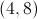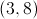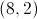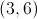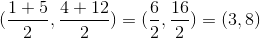# PSAT Math : Geometry

## Example Questions

### Example Question #1 : How To Find The Equation Of A Perpendicular Line

What is the equation for the line that is perpendicular to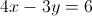through point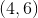?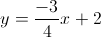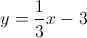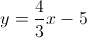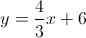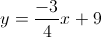Explanation:

Perpendicular slopes are opposite reciprocals.

The given slope is found by converting the equation to the slope-intercept form.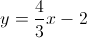The slope of the given line is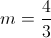and the perpendicular slope is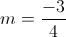.

We can use the given point and the new slope to find the perpendicular equation. Plug in the slope and the given coordinates to solve for the y-intercept.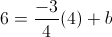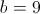Using this y-intercept in slope-intercept form, we get out final equation:.

### Example Question #2 : How To Find The Equation Of A Perpendicular Line

Which line below is perpendicular to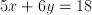?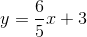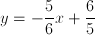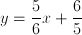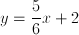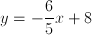Explanation:

The definition of a perpendicular line is one that has a negative, reciprocal slope to another.

For this particular problem, we must first manipulate our initial equation into a more easily recognizable and useful form: slope-intercept form or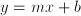.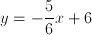According to ourformula, our slope for the original line is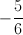. We are looking for an answer that has a perpendicular slope, or an opposite reciprocal. The opposite reciprocal ofis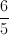. Flip the original and multiply it by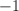Our answer will have a slope of. Search the answer choices forin the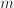position of theequation.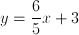is our answer.

(As an aside, the negative reciprocal of 4 is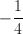. Place the whole number over one and then flip/negate. This does not apply to the above problem, but should be understood to tackle certain permutations of this problem type where the original slope is an integer.)

### Example Question #1 : How To Find The Equation Of A Perpendicular Line

If a line has an equation of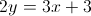, what is the slope of a line that is perpendicular to the line?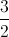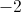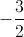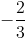Explanation:

Putting the first equation in slope-intercept form yields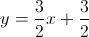.

A perpendicular line has a slope that is the negative inverse. In this case,.

### Example Question #91 : Coordinate Geometry

Which of the following equations represents a line that goes through the pointand is perpendicular to the line?Explanation:

In order to solve this problem, we need first to transform the equation from standard form to slope-intercept form:Transform the original equation to find its slope.First, subtractfrom both sides of the equation.Simplify and rearrange.Next, divide both sides of the equation by 6.The slope of our first line is equal to. Perpendicular lines have slopes that are opposite reciprocals of each other; therefore, if the slope of one is x, then the slope of the other is equal to the following:Let's calculate the opposite reciprocal of our slope:The slope of our line is equal to 2. We now have the following partial equation:We are missing the y-intercept,. Substitute the x- and y-values in the given pointto solve for the missing y-intercept.Add 4 to both sides of the equation.Substitute this value into our partial equation to construct the equation of our line:### Example Question #1 : How To Find The Midpoint Of A Line Segment

A line segment has endpoints (0,4) and (5,6). What are the coordinates of the midpoint?

(0,6)

(2.5,5)

(2.5,-5)

(3,9)

(0,4)

(2.5,5)

Explanation:

A line segment has endpoints (0,4) and (5,6). To find the midpoint, use the midpoint formula:

X: (x1+x2)/2 = (0+5)/2 = 2.5

Y: (y1+y2)/2 = (4+6)/2 = 5

The coordinates of the midpoint are (2.5,5).

### Example Question #1 : How To Find The Midpoint Of A Line Segment

Find the midpoint between (-3,7) and (5,-9)

(-1,-1)

(4,-1)

(1,-1)

(4,-8)

(1,-8)

(1,-1)

Explanation:

You can find the midpoint of each coordinate by averaging them.  In other words, add the two x coordinates together and divide by 2 and add the two y coordinates together and divide by 2.

x-midpoint = (-3 + 5)/2 = 2/2 = 1

y-midpoint = (7 + -9)/2 = -2/2 = -1

(1,-1)

### Example Question #1 : How To Find The Midpoint Of A Line Segment

Find the coordinates for the midpoint of the line segment that spans from (1, 1) to (11, 11).

(5, 5)

(6, 5)

(7, 7)

(6, 6)

(5, 6)

(6, 6)

Explanation:

The correct answer is (6, 6). The midpoint formula is ((x+ x2)/2),((y+ y2)/2) So 1 + 11 = 12, and 12/2 = 6 for both the x and y coordinates.

### Example Question #1 : How To Find The Midpoint Of A Line Segment

What is the midpoint between the points (–1, 2) and (3, –6)?

(–1,2)

(1,–2)

(1,2)

(3,1)

(–2,–1)

(1,–2)

Explanation:

midpoint = ((x1 + x2)/2, (y1 + y2)/2)

= ((–1 + 3)/2, (2 – 6)/2)

= (2/2, –4/2)

= (1,–2)

### Example Question #1 : How To Find The Midpoint Of A Line Segment

A line segment connects the points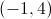and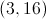. What is the midpoint of this segment?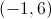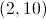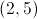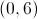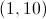Explanation:

To solve this problem you will need to use the midpoint formula: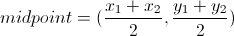Plug in the given values for the endpoints of the segment:and.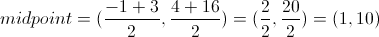### Example Question #6 : How To Find The Midpoint Of A Line Segment

What is the midpoint between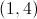and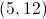?